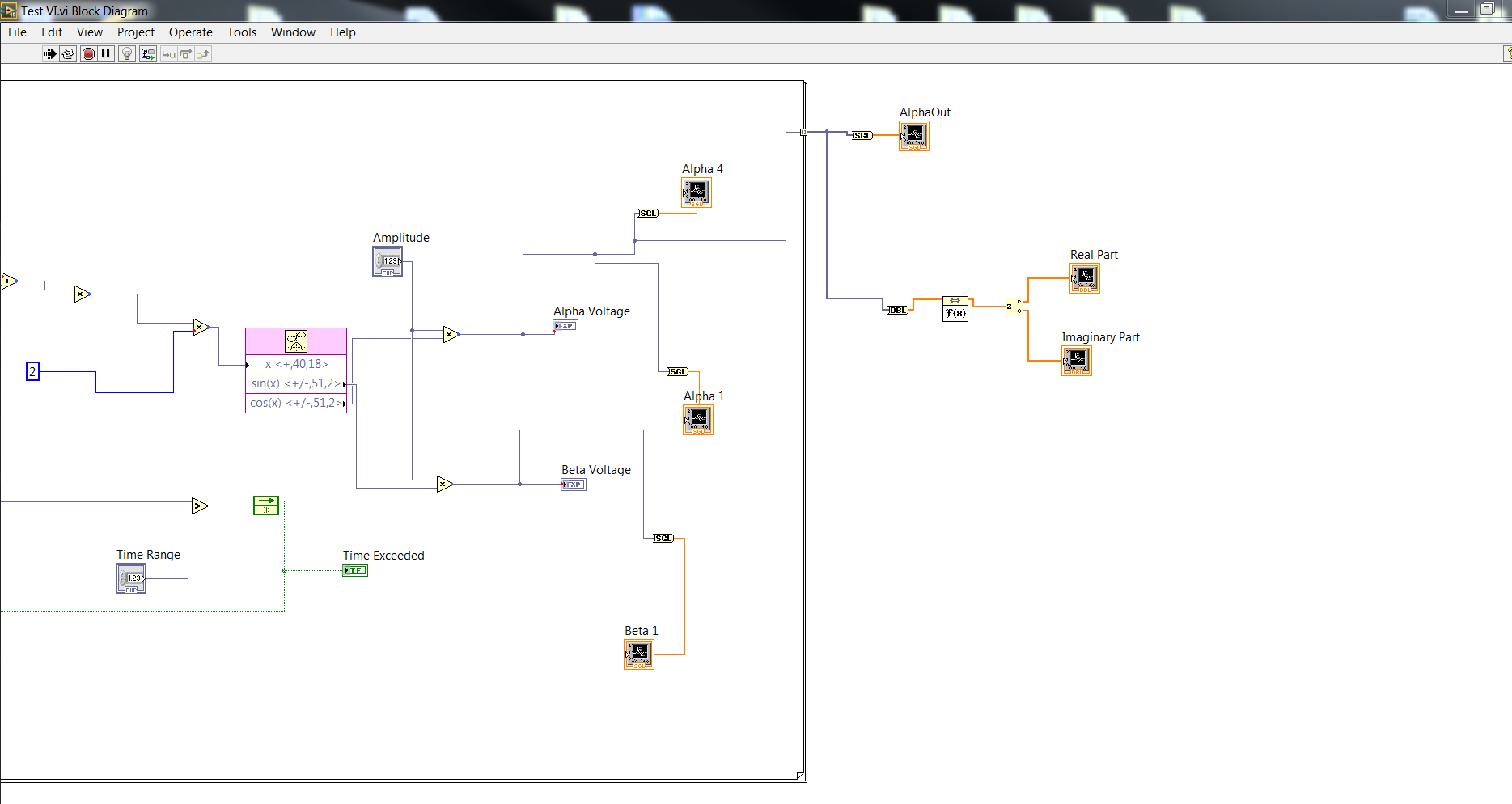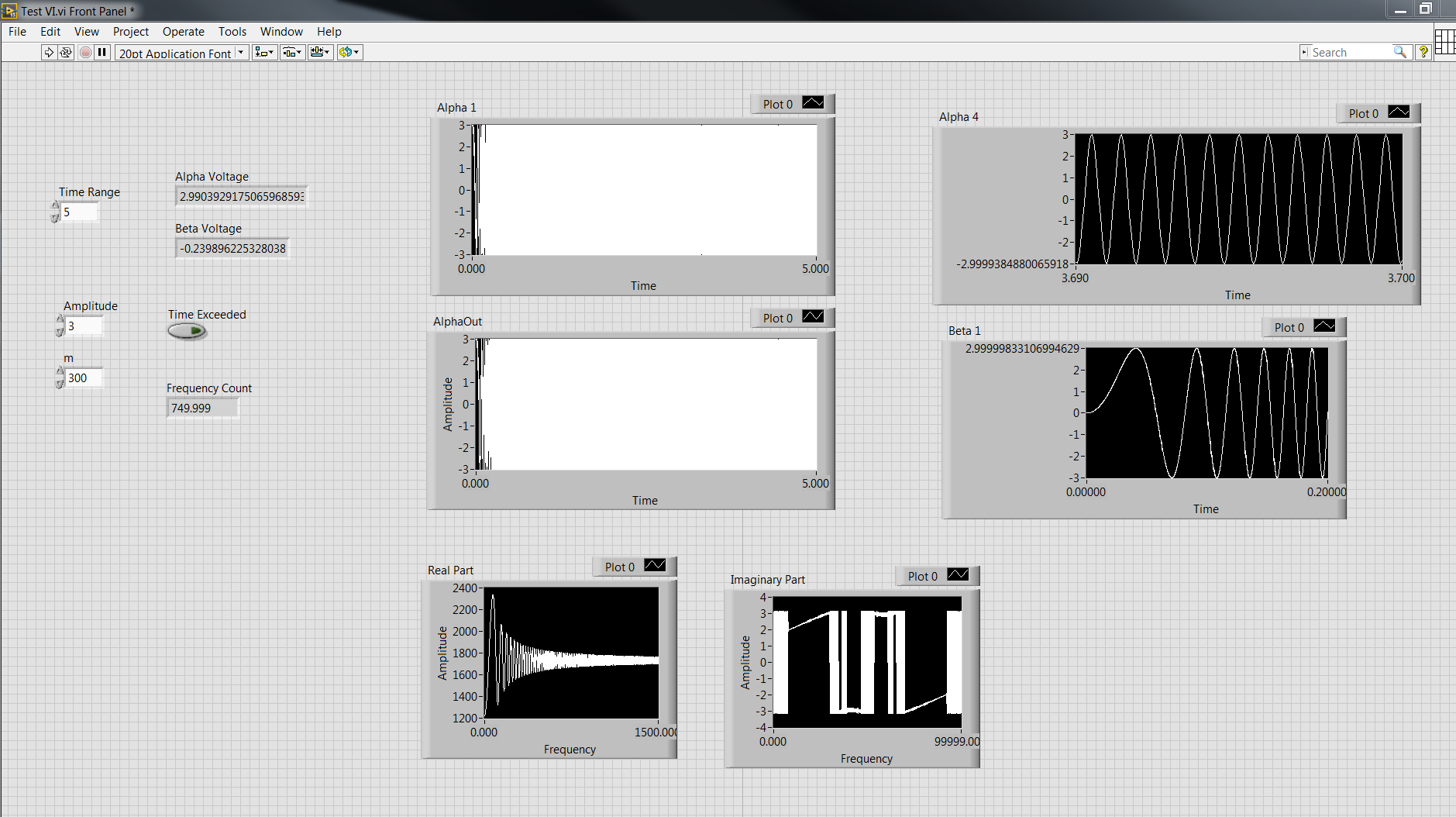## Recommended Posts

I've been using Labview for about 2.5 months. Right now I am working on an already available source code in order to change a few things for the measurements that we want to do.

The problem I have is as follows:

There is a signal in the time domain coming in, that is being transformed through an FFT to the frequency domain (in a for loop). When I plot the signal (in another for loop), the frequency axis is not being displayed properly. how can I make sure that the frequency axis is displayed properly?

Here are screenshots of the block diagram with 1. the time domain plot and 2. the frequency domain plot.

##### Share on other sites

I can't tell much about this becasue I can't see the data and graph on the front panel (FP) and you don't say what is wrong with the frequency axis display, only that you don't find it to be proper. Given that, the first question has to be - how do you know it it isn't proper? Did you check it by some other means? Or is it nonsense? Is the data array transposed?

Nevertheless, I can make general comments. Without wishing to be rude, if you give it data formated the way the plot needs to be, you will get what you want. The reason you don't have what you want is becasue the data is formated wrong. You need to show and explain to get specific help.

If you activate the Contex Help (Ctrl-H in English LabVIEW) and pass your cursor over the icon on the block diagram (BD) the context help will show you the data forms that will produce a graph.

If you just give it 1D data, it will assume a regular unit interval and put all the data on the Y axis.

You get coordiate pairs like this

(0, m0) (u, m1) (2u, m2)...

The waveform data form gives you a method of specifying the regular intervals by providing a starting x value and an interval length. The x axis is then implicit and you only provide the Y data to go with it.

If you give it 2D data it will put up a multiplot with a unit interval between each like pair or more of data.

you get one plot

(0, m0) (u, m1) (2u, m2)...

and another

(0, n0) (u, n1) (2u, n2)...

You must give it two rows of Y data. If you don't want regular intervals between each point -then you need to get involved in an XY plot.

If you give it two columns of Y data, then you need to transpose the array.

Imagine that you gave it 2 data sets with 10 elements each but they were columnar instead of rows, you would get 10 plots with 2 points each. Transpose that and you get 2 plots with 10 points each.

One way to learn this is to create fake data in a constant or control that has a known result and tweak it until the graph does what you want. Then give it real data in the same format.

Mike

##### Share on other sites

Thanks for answering Mike. Here are my answers to some of your questions; below you will find a screenshot of the graphs:

The frequency graphs display on the x-axis the numbers from 0 to 6375 which does not correspond to the frequencies, because the sampling rate of the data is 1024 Hz, which would correspond to frequencies from 0 to 512 (if only half the data were plotted). Furthermore, since I am testing it using some EEG measurement apparatus, measuring nothing more than the 50 Hz buzz of the lamps, I should see the highest peak at 50 Hz and not at around 600. To make sure that my assumptions are correct, I wrote a short matlab script which plots an fft signal. I then loaded it into a mathscript node and let it run. You can see the result on the screenshot below. The 50 Hz are clearly present and also the 150 Hz and 250 Hz harmonics.

I will have to check to see if the data is formatted correctly, but I am pretty sure that it is.

Paul

##### Share on other sites

QUOTE(wiggie @ Jan 16 2008, 09:24 AM)

I will have to check to see if the data is formatted correctly, but I am pretty sure that it is.

Paul

Remeber that it is plotting exactly what you are telling it to. You have to give it the right data in the correct format to get it you show what you want.

If you just give it a 1D array of magnitudes, the graph will simply plot them spaced evenly and apply a defeault increment for the x axis. You need to tell it how to scale the x axis in some way.

The old way was to use the Waveform Data format which is a cluster of the 1D data array, and a scalar each for the X0, inital x value, and the increment for the x axis, dX. The context help for the graph shows you that.

Anytime you have regular x axis increments beteen data a simple 1D array is all you need to produce a plot. Then you can change the plot scale usning the graph properties. You access this by right clicking the graph on the FP and selecting Proerties. Go to the Scale tab and tell it the offset and multiplier. If you leave Autoscale ON then the graph will put the last datum on the right edge of the graph.

Alternately, you can create the Waveform cluster which contains the X0, inital value (offset), and the x increment, dX (multiplier). Then you don't need to change the properties and you can control the offset and multiplier programatically.

I assume you can still create multiplots from wavform data, but they just don't spell it out in the context help anymore (LV keeps changing).

If you send me a set of real data, I can make an example vi. That is actually simpler than trying to verbalize all the permutations. Just take an indicator of the data array, make it default to the current data, drop it in a new vi, name it, and send it to me.

Mike

##### Share on other sites

I have changed the multiplier on the Scale tab of the graph properties from 25 to 2. That made the difference. The data coming in to the graph has 256 samples, which is quite strange. I will play around with the program and see what else I find out. Right now I can see the 50 Hz peak at a value of 50, and that tells me that the frequency axis is correct.

Thank you very much for your help, it's appreciated.

Paul

##### Share on other sites

Hey @mross
I have a very similar issue. Your help  would be greatly appreciated.

I am trying to get the FFT of a 1D array which is basically an excited sine wave (or what many know as a 'chirp').
However, the data points seem to be too large in magnitude. Is there a scaling factor or anything of that sort that I may be missing?
I have made sure that the inputs to the FFT block are what I am expecting and that the FFT is set to process a 1D array.
Since this partial work-product, I am unable to supply the VI but have added extensive pics detailing the issue.

'Alpha 4' shows the chirp mid way and shows the waves are clean and without distortion at a high frequency.
'Beta 1' shows the same thing but at a lower frequency.
'AlphaOut' is the signal that I want and I have probed/zoomed to verify this.
The top 4 graphs are all the same (i.e. a sine wave being excited from 0-1500Hz in 5 secs at a rate of 300Hz/second). All graphs have been zoomed in at different points to show clarity.
The 'Real Part' graph is where I am stuck.
Please let me know if any other info is needed.
Any ideas or help would be greatly appreciated.
Thanks## Join the conversation

You can post now and register later. If you have an account, sign in now to post with your account.×   Pasted as rich text.   Paste as plain text instead

Only 75 emoji are allowed.

×   Your previous content has been restored.   Clear editor

×   You cannot paste images directly. Upload or insert images from URL.

×

×